# Exercise 7.2.9 Let T:V → V be a linear transformation where V is finite dimensional. Show...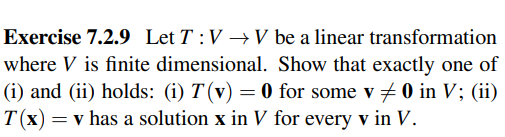Exercise 7.2.9 Let T:V → V be a linear transformation where V is finite dimensional. Show that exactly one of (i) and (ii) holds: (i) T(v) = 0 for some v + 0 in V; (ii) T(x) = v has a solution x in V for every v in V.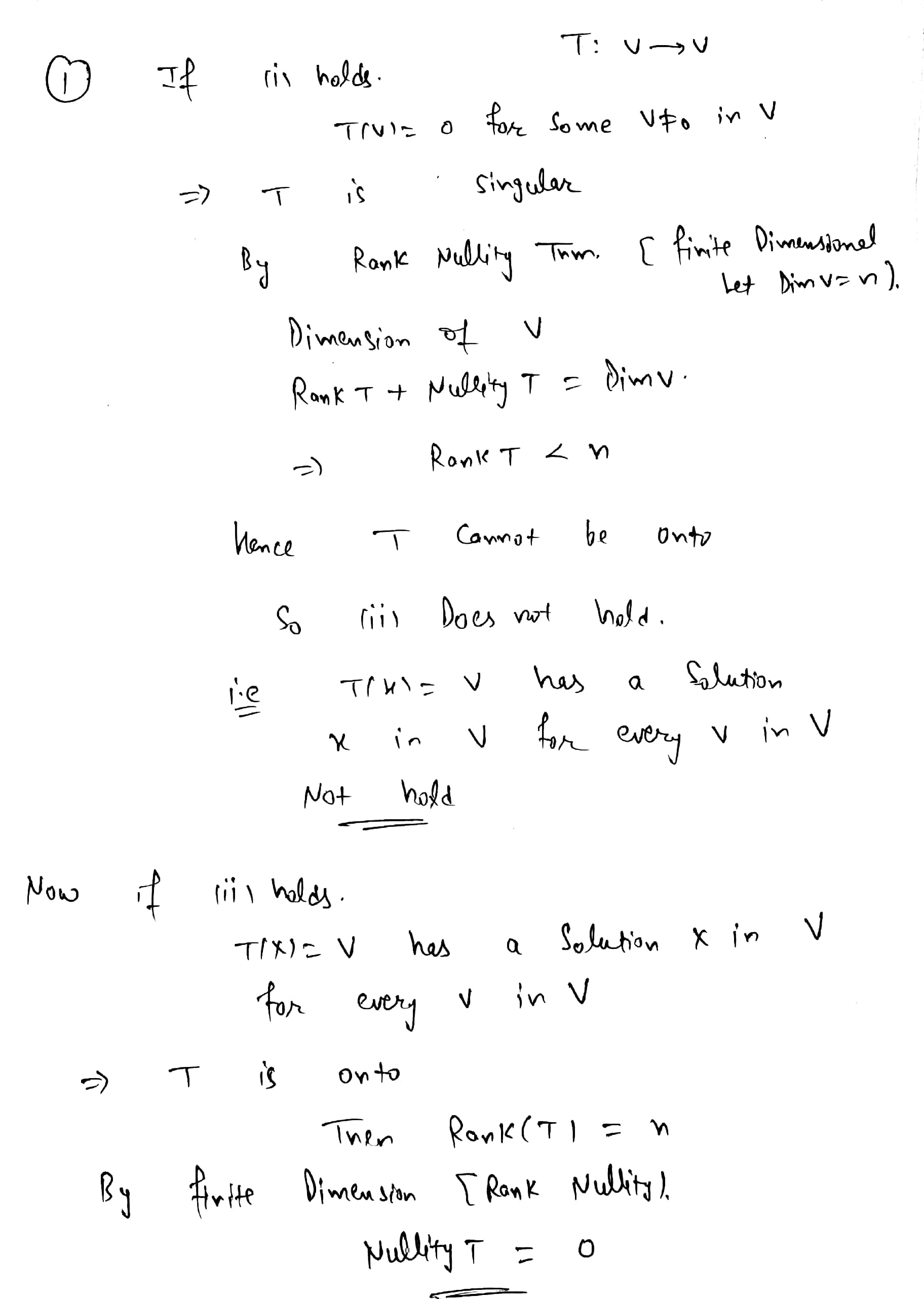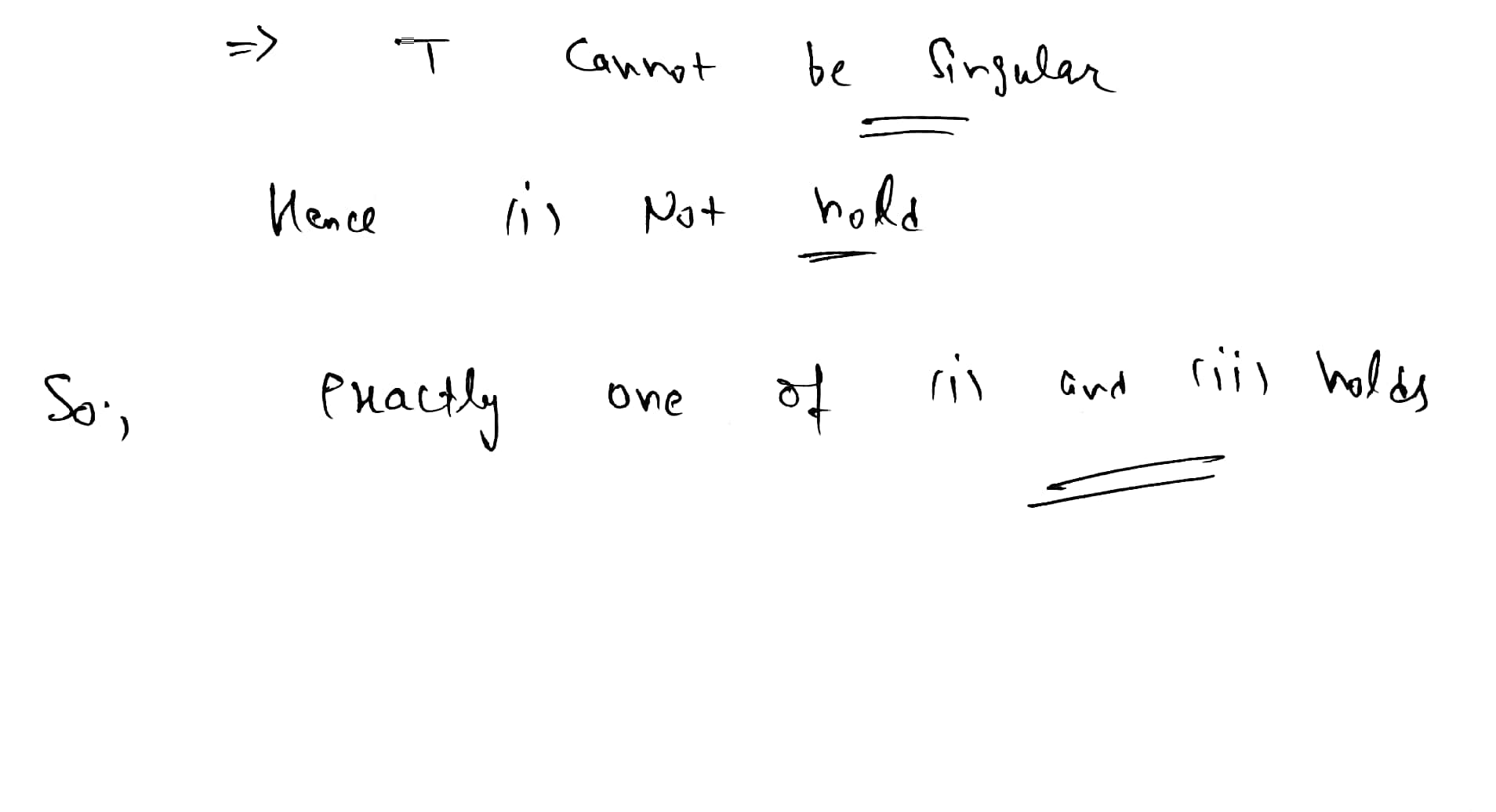##### Add Answer of: Exercise 7.2.9 Let T:V → V be a linear transformation where V is finite dimensional. Show...
Similar Homework Help Questions
• ### Exercise 11.5.9 Let U and V be finite dimensional spaces over F and let θ : U linear map. v be a ...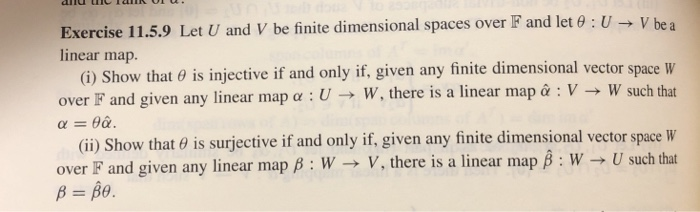Vectors pure and applied Exercise 11.5.9 Let U and V be finite dimensional spaces over F and let θ : U linear map. v be a (i) Show that o is injective if and only if, given any finite dimensional vector space W map : V W such that over F and given any linear map α : U-+ W, there is a linear (ii) Show that θ is surjective if and only if, given any finite dimensional vector space...

• ### Let V and W be two vector spaces over R and T:V + W be a...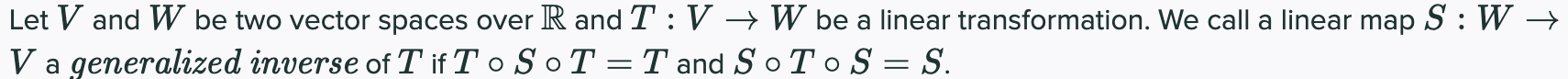Let V and W be two vector spaces over R and T:V + W be a linear transformation. We call a linear map S:W → V a generalized inverse of T if To SoT=T and SoToS = S. If V and W are finite dimensional, show that there exists a generalized inverse of T.

• ### Let V and W be two vector spaces over R and T:V + W be a...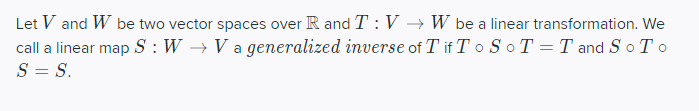Let V and W be two vector spaces over R and T:V + W be a linear transformation. We call a linear map S: W → V a generalized inverse of T if To SOT = T and SoTo S = S. If V and W are finite dimensional, show that there exists a generalized inverse of T.

• ### Let V and W be finite dimensional vector spaces over R and T:V + W be...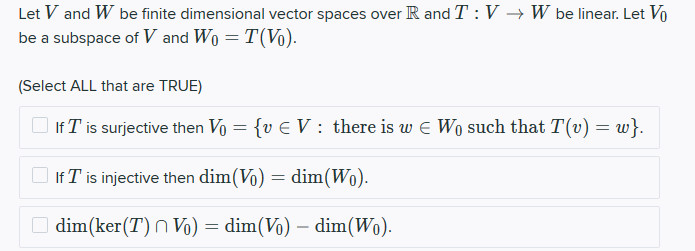Let V and W be finite dimensional vector spaces over R and T:V + W be linear. Let V be a subspace of V and Wo = T(V). (Select ALL that are TRUE) If T is surjective then Vo = {v EV : there is w E Wo such that T(v) = w} If T is injective then dim(VO) = dim(W). dim(ker(T) n Vo) = dim(VO) - dim(Wo).

• ### Let V be a finite-dimensional complex vector space and let T from V to V be...

Let V be a finite-dimensional complex vector space and let T from V to V be a linear transformation. Show that V is the direct sum of U and W where W and U are T-invariant subspaces and the restriction of T on U is nilpotent and the restriction of T on W is an isomorphism.

• ### Let V be a finite-dimensional vector space, and let f :V + V be a linear...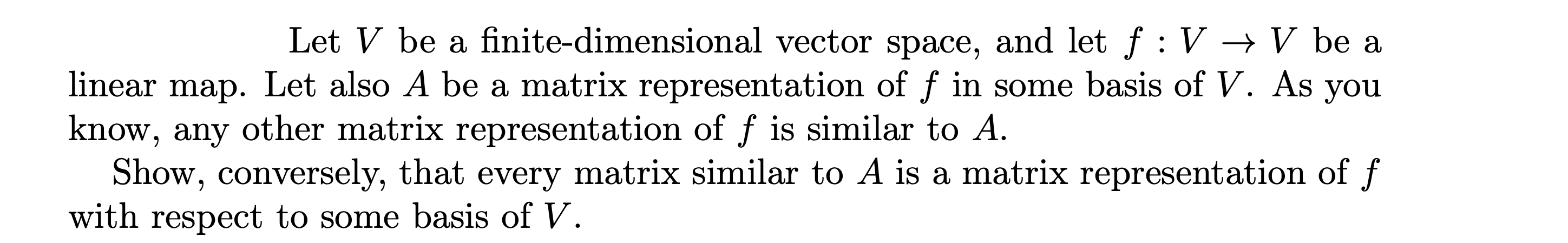Let V be a finite-dimensional vector space, and let f :V + V be a linear map. Let also A be a matrix representation of f in some basis of V. As you know, any other matrix representation of f is similar to A. Show, conversely, that every matrix similar to A is a matrix representation of f with respect to some basis of V.

• ### Let V, W, and U be three finite dimensional vector spaces over R and T:V +...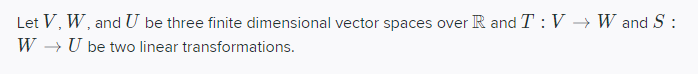Let V, W, and U be three finite dimensional vector spaces over R and T:V + Wand S : W → U be two linear transformations. Show that null(SoT) < null(T) + null(S)

• ### Q10.2 3 Points Let V and W be finite dimensional vector spaces over R and T:V...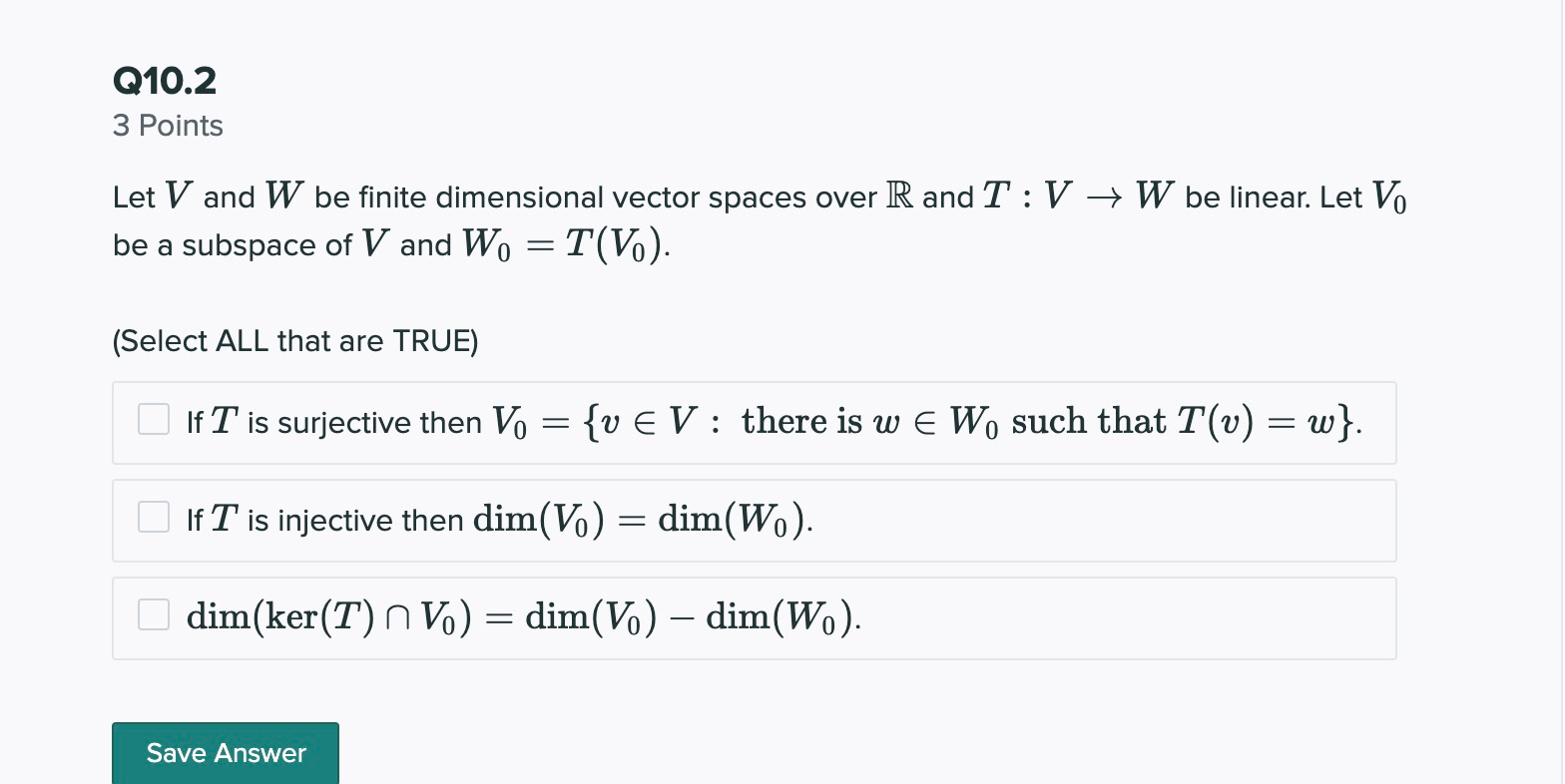Q10.2 3 Points Let V and W be finite dimensional vector spaces over R and T:V + W be linear. Let Vo be a subspace of V and Wo = T(V). (Select ALL that are TRUE) If T is surjective then Vo = {v E V: there is w E Wo such that T(v) = w}. If T is injective then dim(V.) = dim(Wo). dim(ker(T) n ) = dim(V.) - dim(Wo). Save Answer

• ### Let V, W, and U be three finite dimensional vector spaces over R and T:V +...Let V, W, and U be three finite dimensional vector spaces over R and T:V + Wand S : W → U be two linear transformations. Show that rank( ST) > rank(T) + rank(S) - dim(W)

• ### Linear transformation

let U be a subspace of a finite dimensional vector space V.a) show that U = ker T for some linear transformation T : V --> Vb) show that U = im S for some linear transformation S : V --> V

Free Homework App